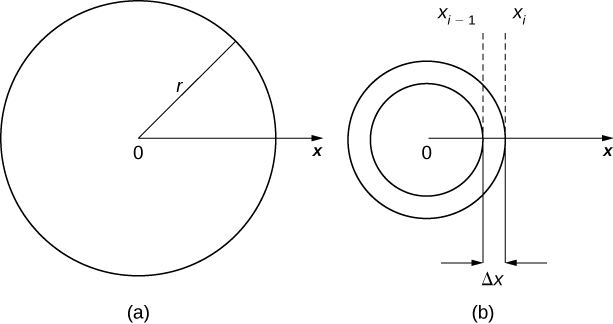# 6.5 Physical applications  (Page 2/11)

 Page 2 / 11(a) A thin disk in the xy -plane. (b) A representative washer.

We now approximate the density and area of the washer to calculate an approximate mass, ${m}_{i}.$ Note that the area of the washer is given by

$\begin{array}{cc}\hfill {A}_{i}& =\pi {\left({x}_{i}\right)}^{2}-\pi {\left({x}_{i-1}\right)}^{2}\hfill \\ & =\pi \left[{x}_{i}^{2}-{x}_{i-1}^{2}\right]\hfill \\ & =\pi \left({x}_{i}+{x}_{i-1}\right)\left({x}_{i}-{x}_{i-1}\right)\hfill \\ & =\pi \left({x}_{i}+{x}_{i-1}\right)\text{Δ}x.\hfill \end{array}$

You may recall that we had an expression similar to this when we were computing volumes by shells. As we did there, we use ${x}_{i}^{*}\approx \left({x}_{i}+{x}_{i-1}\right)\text{/}2$ to approximate the average radius of the washer. We obtain

${A}_{i}=\pi \left({x}_{i}+{x}_{i-1}\right)\text{Δ}x\approx 2\pi {x}_{i}^{*}\text{Δ}x.$

Using $\rho \left({x}_{i}^{*}\right)$ to approximate the density of the washer, we approximate the mass of the washer by

${m}_{i}\approx 2\pi {x}_{i}^{*}\rho \left({x}_{i}^{*}\right)\text{Δ}x.$

Adding up the masses of the washers, we see the mass $m$ of the entire disk is approximated by

$m=\sum _{i=1}^{n}{m}_{i}\approx \sum _{i=1}^{n}2\pi {x}_{i}^{*}\rho \left({x}_{i}^{*}\right)\text{Δ}x.$

We again recognize this as a Riemann sum, and take the limit as $n\to \infty .$ This gives us

$m=\underset{n\to \infty }{\text{lim}}\sum _{i=1}^{n}2\pi {x}_{i}^{*}\rho \left({x}_{i}^{*}\right)\text{Δ}x={\int }_{0}^{r}2\pi x\rho \left(x\right)dx.$

We summarize these findings in the following theorem.

## Mass–density formula of a circular object

Let $\rho \left(x\right)$ be an integrable function representing the radial density of a disk of radius $r.$ Then the mass of the disk is given by

$m={\int }_{0}^{r}2\pi x\rho \left(x\right)dx.$

## Calculating mass from radial density

Let $\rho \left(x\right)=\sqrt{x}$ represent the radial density of a disk. Calculate the mass of a disk of radius 4.

Applying the formula, we find

$\begin{array}{cc}\hfill m& ={\int }_{0}^{r}2\pi x\rho \left(x\right)dx\hfill \\ & ={\int }_{0}^{4}2\pi x\sqrt{x}dx=2\pi {\int }_{0}^{4}{x}^{3\text{/}2}dx\hfill \\ & =2\pi {\frac{2}{5}{x}^{5\text{/}2}|}_{0}^{4}=\frac{4\pi }{5}\left[32\right]=\frac{128\pi }{5}.\hfill \end{array}$

Let $\rho \left(x\right)=3x+2$ represent the radial density of a disk. Calculate the mass of a disk of radius 2.

$24\pi$

## Work done by a force

We now consider work. In physics, work is related to force, which is often intuitively defined as a push or pull on an object. When a force moves an object, we say the force does work on the object. In other words, work can be thought of as the amount of energy it takes to move an object. According to physics, when we have a constant force, work can be expressed as the product of force and distance.

In the English system, the unit of force is the pound and the unit of distance is the foot, so work is given in foot-pounds. In the metric system, kilograms and meters are used. One newton is the force needed to accelerate $1$ kilogram of mass at the rate of $1$ m/sec 2 . Thus, the most common unit of work is the newton-meter. This same unit is also called the joule . Both are defined as kilograms times meters squared over seconds squared $\left(\text{kg}·{\text{m}}^{2}\text{/}{\text{s}}^{2}\right).$

When we have a constant force, things are pretty easy. It is rare, however, for a force to be constant. The work done to compress (or elongate) a spring, for example, varies depending on how far the spring has already been compressed (or stretched). We look at springs in more detail later in this section.

Suppose we have a variable force $F\left(x\right)$ that moves an object in a positive direction along the x -axis from point $a$ to point $b.$ To calculate the work done, we partition the interval $\left[a,b\right]$ and estimate the work done over each subinterval. So, for $i=0,1,2\text{,…},n,$ let $P=\left\{{x}_{i}\right\}$ be a regular partition of the interval $\left[a,b\right],$ and for $i=1,2\text{,…},n,$ choose an arbitrary point ${x}_{i}^{*}\in \left[{x}_{i-1},{x}_{i}\right].$ To calculate the work done to move an object from point ${x}_{i-1}$ to point ${x}_{i},$ we assume the force is roughly constant over the interval, and use $F\left({x}_{i}^{*}\right)$ to approximate the force. The work done over the interval $\left[{x}_{i-1},{x}_{i}\right],$ then, is given by

any genius online ? I need help!!
Pina
need to learn polynomial
Zakariya
i will teach...
nandu
I'm waiting
Zakariya
evaluate the following computation (x³-8/x-2)
teach me how to solve the first law of calculus.
what is differentiation
f(x) = x-2 g(x) = 3x + 5 fog(x)? f(x)/g(x)
fog(x)= f(g(x)) = x-2 = 3x+5-2 = 3x+3 f(x)/g(x)= x-2/3x+5
diron
pweding paturo nsa calculus?
jimmy
how to use fundamental theorem to solve exponential
find the bounded area of the parabola y^2=4x and y=16x
what is absolute value means?
Chicken nuggets
Hugh
🐔
MM
🐔🦃 nuggets
MM
(mathematics) For a complex number a+bi, the principal square root of the sum of the squares of its real and imaginary parts, √a2+b2 . Denoted by | |. The absolute value |x| of a real number x is √x2 , which is equal to x if x is non-negative, and −x if x is negative.
Ismael
find integration of loge x
find the volume of a solid about the y-axis, x=0, x=1, y=0, y=7+x^3
how does this work
Can calculus give the answers as same as other methods give in basic classes while solving the numericals?
log tan (x/4+x/2)
Rohan
Rohan
y=(x^2 + 3x).(eipix)
Claudia
Ismael
A Function F(X)=Sinx+cosx is odd or even?
neither
David
Neither
Lovuyiso
f(x)=1/1+x^2 |=[-3,1]
apa itu?
fauzi
determine the area of the region enclosed by x²+y=1,2x-y+4=0
Hi
MP
Hi too
Vic
hello please anyone with calculus PDF should share
Which kind of pdf do you want bro?
Aftab
hi
Abdul
can I get calculus in pdf
Abdul
explain for me
Usman
okay I have such documents
Fitzgerald
Hamza

#### Get Jobilize Job Search Mobile App in your pocket Now!By OpenStaxBy John GabrieliBy Ellie BanfieldBy Angela JanuaryBy Alec MoffitBy OpenStaxBy Hannah ShethBy Anonymous UserBy OpenStaxBy David Corey